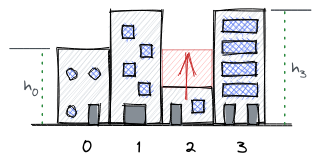# Buildingsshowmic96Codeware19: Intra AUST Pr...
Limits 1s, 512 MB

There are $n$ buildings in a row. The height of the $i$-th building is $h_i$.You have $৳k$. It is the amount of money you have. With $৳1$ you can increase the height of a building by 1. So you want to spend $৳k$ in such a way that after spending $৳k$ the height of the lowest building will be maximized.

## Input

The first line of the input will consist of two integers $n$ ($1 ≤ n ≤ 10^5$) the number of buildings and $k$ ($0 ≤ k ≤ 10^9$) the amount of money you have. The next line will consist of $n$ integers the height of the buildings ($0 ≤ h_i ≤ 10^9$).

## Output

Print a single integer the height of the lowest building.

## Samples

InputOutput
5 10
1 2 3 4 5

5

InputOutput
1 10
10

20uDebug

### Submit